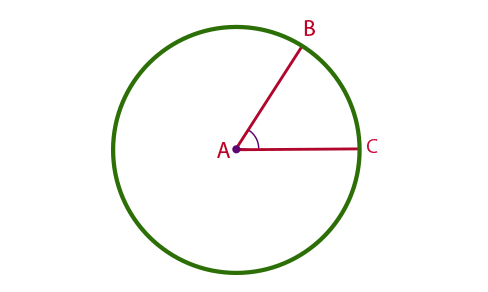# Central Angle of a Circle Formula

The angle between two radii of a circle is known as the central angle of the circle. The two points of the circle, where the radii intersect in the circle (Note – The other end of the radii meets at the centre of the circle), forms a segment of the Circle called the Arc Length.Formula to Find the Central Angle of a Circle –
$Central\;Angle\;\theta =\frac{Arc\;Length\times 360^{o}}{2\pi r}$

### Solved Examples

Example 1: Find the central angle, where the arc length measurement is about 20 cm and the length of the radius measures 10 cm.

Solution:

Given,

r = 10 cm

Arc length = 20 cm

The formula of central angle is,

Central Angle

$$\begin{array}{l}\theta\end{array}$$
=
$$\begin{array}{l}\frac{Arc Length \times 360^{o}}{2 \times\pi \times r}\end{array}$$

Central Angle

$$\begin{array}{l}\theta\end{array}$$
=
$$\begin{array}{l}\frac{20 \times 360^{o}}{2 \times 3.14 \times 10}\end{array}$$

Central Angle

$$\begin{array}{l}\theta\end{array}$$
=
$$\begin{array}{l}\frac{7200}{62.8}\end{array}$$
= 114.64°

Example 2: If the central angle of a circle is 82.4° and the arc length formed is 23 cm then find out the radius of the circle.

Solution:

Given,

Arc length = 23 cm

The formula of central angle is,

Central Angle

$$\begin{array}{l}\theta\end{array}$$
=
$$\begin{array}{l}\frac{Arc\;Length \times 360^{o}}{2\times\pi \times r}\end{array}$$

82.4° =

$$\begin{array}{l}\frac{23 \times 360^{o}}{2\times\pi \times r}\end{array}$$

82.4° =

$$\begin{array}{l}\frac{8280}{6.28\times r}\end{array}$$

r=

$$\begin{array}{l}\frac{8280}{6.28\times 82.4}\end{array}$$

r = 16 cm